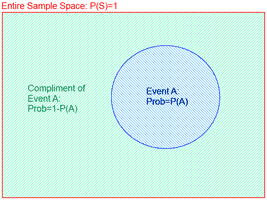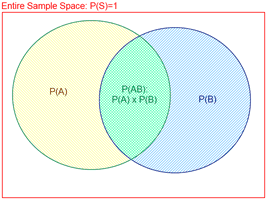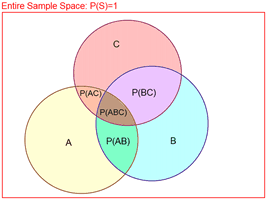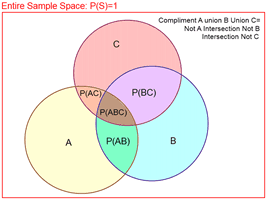In the first half of this article, I touched on why I thought understanding probability was integral to playing, running, and especially designing role playing games and then presented the underpinnings of basic probability and counting methods. This article takes up where that one left off and dives straight into the math of probability.

Last time we looked at how to build base probabilities from the simple sample space. This time we’re taking a look at the properties of the resultant probabilities and how they can be transformed into more complex and useful forms.

P(Sample Space)=1
The Sample Space is the collection of all possible events. Thus, P(Sample Space) is the probability that some combination of possible events will happen, including the probability that nothing will happen at all if it’s possible. As such, it’s probability is 1.

The probability that we roll a number between 1 and 20 on a d20 is 1

P(Empty Set)=0
The Empty Set is the collection of all impossible events. Thus, P(Sample Space) is the probability that something impossible will happen. As such it’s probability is 0.

The probability we roll the letter Q on a D4 is 0

P(Disjoint Event A OR Disjoint Event B)=P(A)+P(B)
If two or more events are disjoint (they share no common outcomes) the Probability of their Union (one OR the other) is equal to the Sum of their probabilities. This is called “Countable Additivity”.

The probability we roll either a 2 OR an odd number on a d6 is P(2)+P(odd). Since P(2)=1/6 and P(odd)=1/2, P(2 Union odd)=P(2)+P(odd)=1/6+1/2=2/3. This works because 2 and the set of odd numbers are disjoint. That is: it’s impossible to roll both a 2 and an odd number on the same die on the same throw.P(A Compliment)=1-P(A)
The Probability of the compliment of event A (the set of outcomes that includes all outcomes NOT in the original event) is equal to 1-P(A). Since Event A and it’s compliment are disjoint (they share no outcomes) they have Countable Additivity. Since together they include the entire Sample Space (which has a probability 1) their probabilities must sum to 1, and
P(A Compliment) must equal 1-P(A).

The probability of rolling a 4 or a 6 on a d6 is the compliment of the probability we calculated in the previous example. Thus P(4 union 6)=1-P(2 Union odd)=1-2/3=1/3.

P(Independent Event A AND Independent Event B)=P(A)*P(B)If two or more events are independent (the outcome of any one does not effect the probabilities of the outcome of any others) the probability of their intersections (that they BOTH happen) is equal to the product of their probabilities. Probabilities of intersections are noted P(AB). If the probability of the two events are NOT independent, this property doesn’t hold. Instead, probabilities of intersections must be calculated with counting methods, by reversing the formula for conditional probability (discussed below) or by breaking them into unions of probabilities that are independent.

The probability of rolling an 18 on 3d6 is P(6 intersection 6 intersection 6). Since P(6)=1/6 and each die roll is independent of the others, P(6 intersection 6 intersection 6)=P(6)*P(6)*P(6)=1/6*1/6*1/6=1/216 (less than half a percent), which is why it annoys the piss out of me when players belly up to my table with multiple 18s and try to pretend they rolled them fairly.P(A Union B)=P(A)+P(B)-P(AB)
The formula for the probability of a union of non-disjoint events is similar to the formula for the union of disjoint events, but since non-disjoint events include intersections, the formula must be corrected to account for double counting. The formula for only two events is P(A)+P(B)-P(AB). A general formula also exists for any number events (the diagram to the right shows three events).
The general formula is:Â  P(union of any number of events)=the sum of the probabilities of each event — the sum of the probabilities of every combination of two events + the sum of the probabilities of every combination of three events — the sum of the probabilities of every combination of four events … and so on alternating the sign and increasing the number of events each time until you subtract or add the intersection of all events.
Thus, the formula for the above diagram is P(A Union B Union C)=[P(A)+P(B)+P(C)]-[P(AB)+P(AC)+P(BC)]+[P(ABC)]

Rolling an even number or a number higher than 3 on a d6 is P(even Union 4+). Since these are NOT disjoint, P(even Union 4+)=P(even)+P(4+)-P(even intersection 4+). P(even)=1/2, P(4+)=1/2, P(even intersection 4+)=1/3. Thus, P(even Union 4+)=1/2+1/2-1/3=2/3

P(A given that we know B has happened)=P(AB)/P(B)Sometimes we know an event has occurred and we want to re-evaluate the probability of another event in light of what we now know. Remember that a probability can be thought of as a proportion between the event in question and the sample space. similarly to how P(A)=|A|/|S| when evaluating counting methods. Looking at the diagram to the right we can visualize the probability of event A, given that event B has occurred as the probability of Event “A and B both occur” in the sample space of “B occurs”. This makes it more obvious why P(A given B has occurred)=P(A Intersection B)/P(B).
P(A given B has occurred) is usually noted P(A|B) and read as Probability A, given B.
Note that if you already know P(A|B) and P(B), this formula can be used to find P(AB).
If A and B are independent, P(A|B)=P(A).

John is using a special ability to do extra damage the more successes he gets on his attack roll. We know that he has a 1/2 chance of hitting AND killing the foe, and he had a 7/10 chance to hit the foe. He’s rolled his attack roll and hit! Before damage could be rolled, the GM called a smoke break. The guy to your left just bet you \$10 that John won’t roll enough damage to kill the enemy. Is that a smart bet? We know P(Kill intersection Hit)=1/2 and P(Hit)=7/10. P(Kill given Hit)=P(Kill intersection Hit)/ P(Hit)=(1/2)/(7/10)=5/7. So John has a very good chance of killing the enemy, and you should probably take that bet.

P(at least one)The probability of unions of groups of non-disjoint events, which we calculated above can be used in situations when you want to know the probability that at least one of a group of events meets with some success condition. In these cases, the size of the group events is often large and the formula involved can get very cumbersome. If the events are independent, however it is often easier to calculate the probability of the compliment of the union and simply subtract that value from one to get the probability of the union.

You have a pool of five d10 and need to roll a 6 or better on at least one of them to succeed. You could use the formula for the probability of unions to determine your chance of success, but that would be very cumbersome. Instead, you can use the formula for calculating independent intersections to find the probability that you get no successes, which is the compliment of at least one success. Since P(Fail on a die)=1/2, P(fail on all dice)=P(intersection of all failing)=(P(a die fails))^5=(1/2)^5=1/32, so your chance of success is 31/32.

The Binomial Formula
By far the most useful formula for die probabilities is the binomial formula. It calculates the probability of achieving x successes given n tries with each try having p chance of success. This is the formula to calculate successes with any type of die pool system. P(X successes)=n!/(x!*(n-x!)) * p^x * (1-p)^(n-x)

As above, you have a pool of five dice, with a 1/2 chance of success of each die, but this time we need 3 or more successes.Â  P(x=3)=5!/(3!*(5-3!)) * (1/2)^3 * (1-(1/2))^(5-3)=5/16, P(x=4)=5!/(4!*(5-4!)) * (1/2)^4 * (1-(1/2))^(5-4)=5/32, P(x=5)=5!/(5!*(5-5!)) * (1/2)^5 * (1-(1/2))^(5-5)=1/32, so P(3+)=5/16+5/32+1/32=1/2

Putting it all Together
You may have already figured out that the binomial formula is actually just a counting method and a formula mashed together. n!/(x!*(n-x!)) is the number of arrangement of x successes and n-x failures that exist, p^x is the probability of the intersection of those independent successes, and (1-p)^(n-x) is the probability of the intersection of the remaining independent failures, all tied together with the multiplication rule and intersection formulas. There’s nothing stopping you from creating your own similar formulas with the tools available.

Further Information
These two articles are only the very surface of probability, but anything further is beyond the scope of an RPG blog. If you’re interested in more you’ll have to hunt down other books and resources.